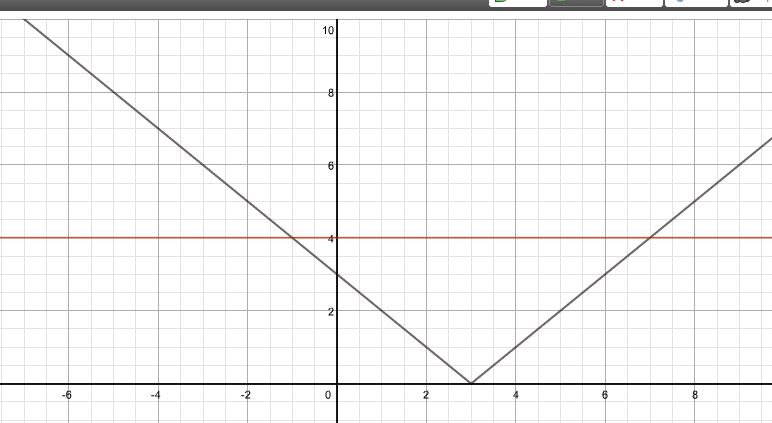# Calculators

Whether you want to work out some simple calculations or compute the eigenvalues and eigenvectors of a matrix there is probably a free online calculator to do the job for you!WolframAlpha is so powerful (and not only for Mathematics) it deserves pages of its own. There are several links available and also slideshows to help you learn to use WolframAlpha. (WolframAlpha is still entirely free to use and so useful for teachers and students, though a new Pro option is now also available).

Using WolframAlpha widgets makes it possible to set up widgets for repeated use, older students for example need to solve absolute value equations.When I find a new online calculator I like I include it in another blog: Mathematics Calculators and Tools so that the collection is together.

To highlight a few of the calculators I have used recently. For students studying quadratic equations this calculator which shows the use of the quadratic formula from Math Warehouse is very useful as it shows full working on how to use the formula.The graphing calculator I return to again and again is the Desmos graphing calculator, for example I was recently studying the modulus function with my sixth form students, looking at modulus equations and inequalities a picture speaks a thousand words! (Just type y=abs…)When marking the Further Mathematicians’ Decision Mathematics paper I found Stefan Waner’s Simplex Tool very useful,The tableaus are shown in full.

1.Colleen Young